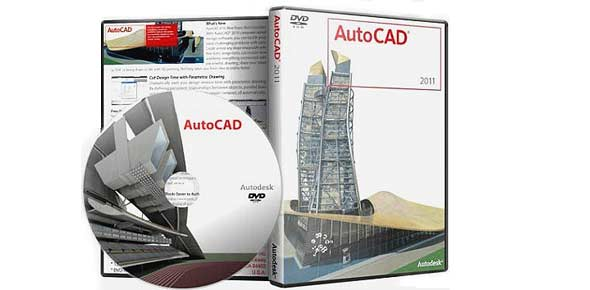# CAD Modelling

25 Questions | Total Attempts: 242SettingsAGRONA 2K15INSTRUCTION:ALL THE QUESTIONS ARE MULTIPLE CHOICESONCE THE QUESTIONS ARE ANSWERED CANNOT BE MODIFIEDDURATION OF THE TEST IS 20 MINUTESDON'T CLOSE THE RESULT PAGE,IF CLOSED YOU WILL BE DISQUALIFIEDAFTER COMPLETION INFORM IMMEDIATELY  TO THE CO-ORDINATORSALL THE BEST

Questions and Answers
• 1.
A drawing giving details about size tolerances heat treatment, etc known as
• A.

Production drawing

• B.

Assembly drawing

• C.

Exploded drawing

• D.

Machine drawing

• 2.
Grid display on the screen with in default position
• A.

Has fixed an equal distance in X and Y direction

• B.

Can have different distances in X and Y directions at 45 degree

• C.

Can have different distances in X and Y direction (or) 30 degree

• D.

Can have different distances in X and Y direction at any angle

• 3.
AUTOCAD environment setting can be seen by command
• A.

OSNAP

• B.

DDRMODES

• C.

STATUS

• D.

SNAP

• 4.
French curves are used for drawing
• A.

Straight lines

• B.

Non-circular lines

• C.

Special type of lines

• D.

Circular shapes

• 5.
Length/width ratio of drawing sheet is
• A.

2:1

• B.

1.515:1

• C.

1.414:1

• D.

1.25:1

• 6.
An extension line is used to show
• A.

An extension part

• B.

The size of an object while dimensioning

• C.

Extra line projection out of the line

• D.

An extrapolated continuous full line

• 7.
Morse taper is used to define
• A.

Differences in diameter per unit length

• B.

Angle of a line

• C.

Code used in dimensioning

• D.

None of above

• 8.
Symbol  φ before a dimension value means
• A.

Diameter

• B.

Non-frictional

• C.

Spherical diameter

• D.

Taper

• 9.
Chain dimensioning is done where there are
• A.

Many same sized links

• B.

Many dimensions in one line

• C.

Overlapping parts

• D.

End points that are off line

• 10.
A line dimensions are used to
• A.

Draw inclined lines

• B.

Dimension angles

• C.

Align dimension line along the line

• D.

Align dimension along horizontal or vertical lines

• 11.
The number of views or the graphic projection is
• A.

Three

• B.

two

• C.

Four

• D.

Depends upon the shape of object

• 12.
In orthographic projection the rays are assumed to
• A.

Diverge from station point

• B.

Converge from station point

• C.

Be parallel

• D.

Make an angle of 30degree

• 13.
Front view of a circle  at 45 degree to vertical plane will be
• A.

Circle

• B.

Ellipse

• C.

Oval

• D.

Line

• 14.
Sectional view are drawn to
• A.

Show internal details

• B.

Section the object in 2 halves

• C.

Section the object in one garter two halves

• D.

Hide external details

• 15.
Which statement is true for cutting plane?
• A.

It is always in straight line

• B.

Its axis depends upon the shape of object

• C.

It is always in center of object

• D.

It makes an angle with the object axis

• 16.
Very thin object in selection is shown by
• A.

Thin lines at 45°

• B.

Lines at 10°

• C.

Vertical lines

• D.

Fully darkened

• 17.
Hatching means
• A.

Lines at regular interval

• B.

Repeated pattern at regular interval

• C.

Repeated pattern at regular interval in a boundary

• D.

Solid filled up area

• 18.
Auxiliary view is drawn to show
• A.

A inclined surface

• B.

Complicated surface

• C.

Actual shape of surface which otherwise is illusive

• D.

Big parts

• 19.
Auxiliary views is drawn
• A.

Opposite to front view

• B.

Parallel to inclined plane

• C.

Opposite to top view

• D.

On the other side of the side view

• 20.
Reverse isometric views is drawn by
• A.

Seeing the object in reverse direction

• B.

Putting the object upside down

• C.

Shifting the plane in front of object

• D.

Changing viewing direction from top view to bottom

• 21.
Section lines in sectional isometric views are drawn at
• A.

45°

• B.

60°

• C.

135°

• D.

30°

• 22.
Four centre methods is used to draw
• A.

Quadrant of a circle

• B.

Elliptical arc

• C.

Iso circle

• D.

Square

• 23.
Angle of isometric grid is
• A.

30°

• B.

45°

• C.

60°

• D.

90°

• 24.
For perspective views a vanishing point is considered at
• A.

infinity

• B.

30° to object at any distances

• C.

45° to object at any distance

• D.

Some suitable distance and angle

• 25.
The term cone angle is used in perspective views for
• A.

Triangular objects

• B.

Conical objects

• C.

For any shape of object

• D.

Rectangular objects

Related Topics